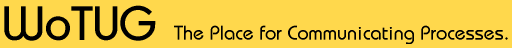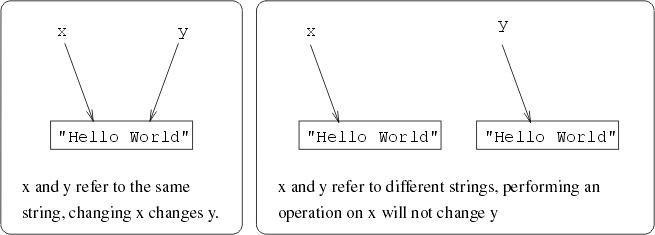## Dynamic string library - using User Defined Operators

James Moores, Computing Lab, University of Kent, UK

26th March 1997

This document is intended to provide a guide to using the dynamic string library supplied as an example usage of the new user defined operators.

Obvious warning: The occam compiler cannot check the usage and aliasing of these dynamically created data types -- they live in the "C" world.

### Overview

The library first defines a new data type -- `DSTRING`, which is a reference to a string. It also provides a set of fuctions for creating and deleting the strings that the variables of this new `DSTRING` type refer to; a string copy function, a set of comparason operators, a concatination operator, length function, printing function, and procedure to convert the new string format back into occam strings.

### Declaration and creation of strings

To declare a new string, all that is required is:

```  DSTRING x:
```
This variable, however does not refer to anything at the moment, so a function must be called to create a string (allocate its memory dynamically) which takes an array of bytes (a standard occam string) and returns a reference to the string created:
```  DSTRING x:
SEQ
x := new.dstring("Hello World*c*n")
```

### Assignment and copying strings

Assigning variables of type `DSTRING` to each other will simply copy the reference to the string:

```  DSTRING x,y:
SEQ
x := new.dstring("Hello World*c*n")
y := x
```
both `x` and `y` now refer to the same string -- containing `"Hello world"`. This means that if an operation is performed on string `x`, string `y` will also have been changed. To make an independent copy of a string, use `copy.dstring`:
```  DSTRING x,y:
SEQ
x := new.dstring("Hello World*c*n")
y := copy.dstring(x)
```
x and y are now independent.

### Deallocation of strings

Strings can be deallocated (destroyed and the memory returned to the heap) using:

```  DSTRING a:
SEQ
a := new.dstring("Hello")
delete.string(a)
```
which should always be done when strings are no longer useful, or the variables holding string pointers are about to go out of scope - otherwise the memory will be ``lost''.

### Conversion to occam strings

There will often be the need to convert a `DSTRING` to an occam format string (array of `BYTE`s). This is done using the routine `oc.string`; note that the array of `BYTE`s passed to it should be big enough to hold the string referenced by the string variable, but if it is insufficient, then the array is filled to capacity. If the array is too big (as will often be the case), then the rest of the array is padded with zero bytes (which will, of course be ignored if the array is 'printed' using the standard C functions).

```  DSTRING a:
BYTE buf:
SEQ
a := new.dstring("Hello")
oc.string(a, buf)
-- buf now contains "Hello\0\0\0\0..." (in C speak)
```

### Boolean comparison operators

The library provides all of the boolean comparison operators on strings. Comparison is done on the basis of lexical equivalence:

```  DSTRING x,y:
SEQ
x := new.dstring("aaaaaa")
y := new.dstring("aaaaab")
IF
(exp)
```

where the exp and its values for the above `x` and `y` are:

 exp Value Comment x = y FALSE x and y are lexically equivalent x < y TRUE x is lexically less than y x > y FALSE x is lexically greater than y x <= y TRUE x is lexically less than or equal to y x >= y FALSE x is lexically less than or equal to y x <> y TRUE x is not equal to y

they are based on the result of the call strcmp(s1,s2) in the C world.

### Reference vs Lexical equivalence

There is a reference equivalence operator `==` which returns true if both the string variables refer to at the same string (See Figure 1)Figure 1: Aliasing with string references

```  DSTRING a,b,c:
SEQ
a := new.dstring("Hello")
b := new.dstring("Hello") -- could be copy.dstring(a)
c := a                    -- c is now an alias for a
IF
(exp)
...
```

where exp and its values for the above program are:

 exp Value Comment a = b TRUE a and b are lexically equivalent a = c TRUE a and b are lexically and reference equivalent, but only lexical equivalence tested here a == b FALSE lexically, but not reference equivalent a == c TRUE reference and lexically equivalent, but only reference equivalence tested here

The concatenation operator is `++` and is used as follows:

```  DSTRING a,b,c:
SEQ
a := new.dstring("Hello")
b := new.dstring(" world")
c := a ++ b
```

`c` now contains the string `"Hello world"`; it is worth noting that this creates a new string holding the union of the two operands, not a modified copy of either `a` or `b` (ie they are left as they were). However, care must be taken not to do the following:

```  DSTRING a,b:
SEQ
a := new.dstring("Hello")
b := new.dstring(" world")
a := a ++ b
```

This is legal occam, but the string that `a` originally referred to is lost, ie we have memory leakage. This will also be a problem if concatenations are nested eg:

```  DSTRING a,b,c,d:
SEQ
a := new.dstring("Hello")
b := new.dstring(" world")
c := new.dstring(" at large")
d := (a ++ b) ++ c
```

The resulting intermediate string reference from evaluating ```(a ++ b)``` will be lost and cause memory leakage. This is really caused by, and the price to be paid for, allowing (in fact by tricking the occam compiler by using calls to C/assembler code it cannot check) expressions to cause side effects, in this case the allocation of memory from the heap.

### Length function

The `dstring.length` function returns the length of the given string:

```  INT length:
DSTRING a:
SEQ
a := new.dstring("Hello")
length := dstring.length(a)
-- length now equals 5
```

it will return the length as defined by the C function strlen(s), which is "the number of characters in s, not including the null-terminating character.".

### A printing function

A simple printing function is provided for convenience:

```  PROC test (CHAN OF BYTE in,out,err)
DSTRING a:
SEQ
a := new.dstring("Hello")
print.dstring(out,a)
```

which will place the string referred to by `a` on the standard output. Please note that the channel passed is currently ignored and stdout is always used.

### Installation and use

The example directory examples/udo contains the source for the library:

• `dstring.occ` -- the occam interface (visible to code) containing the user defined operator functions (eg `++`, `=`, `==`, `!=` etc.) normal functions (eg `new.dstring`) and procedures (eg `oc.dstring`)
• `c_dstring.c` -- the C functions called by the above (and their interfaces, which convert between calling conventions)
• `conv.occ` -- two occam routines that convert between the endianess of the transputer world, and that of the native world (which is what C works in)

These can be built using the given Makefile - just type ``make''. This will compile and build a native code library libdstring.a and an occam module dstring.tco.

If a ``make install'' is done, the library elements will be copied into the default KROC library area and in that case programs can be compiled using:

```  kroc -X2 prog.occ -ldstring
```

the -X2 flag turns on the experimental user defined operators.

Note that if you don't want to install the library you will have to use the -L flag of kroc to indicate the directory containing the library. The -L flag can be dropped if the environment variable OCSEARCH is set to include this directory.

At the start of programs using this library you must include the `DSTRING` definitions, inline `FUNCTION`s and reference the occam module dstring.tco:

```#INCLUDE "dstring.inc"
#INCLUDE "dstring.oci"
#USE "dstring.tco"
PROC main.code (...)
...  use DSTRING here
:
```

### Bugs

ofa-bugs@kent.ac.uk
although please note that we cannot guarantee support.

Dynamic string library - an example of User Defined Operators

This document was originally generated using the LaTeX2HTML translator Version 96.1 (Feb 5, 1996) Copyright © 1993, 1994, 1995, 1996, Nikos Drakos, Computer Based Learning Unit, University of Leeds.

The command line arguments were:
latex2html -split 0 dstring.tex.

The document was then edited by Dave Beckett to be better HTML.